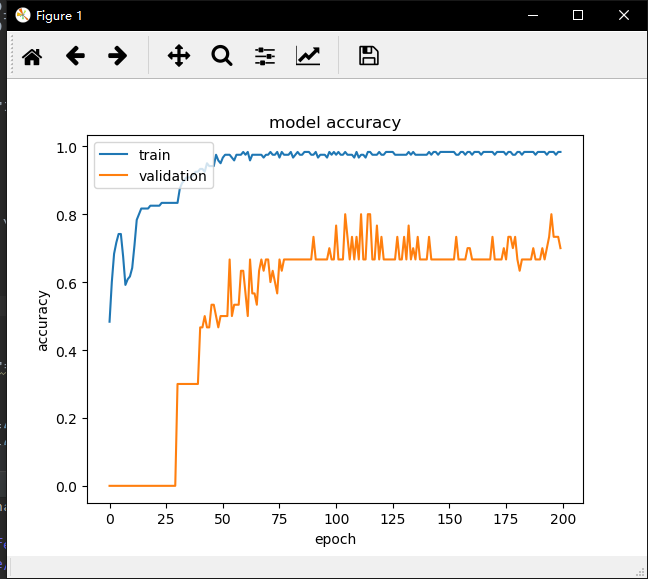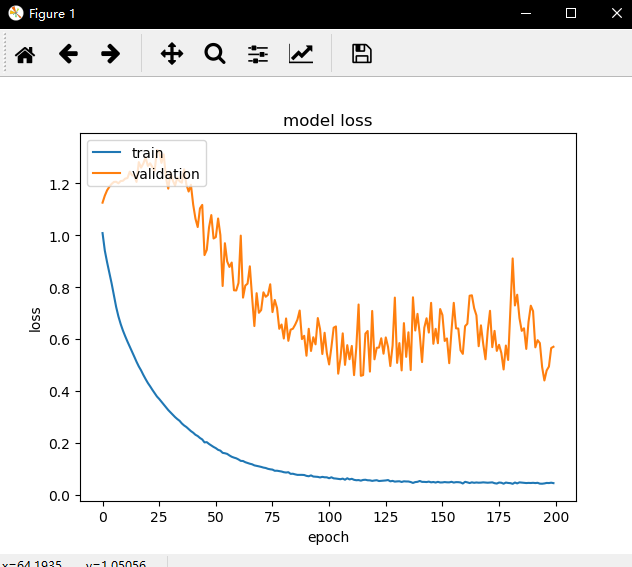• 深度学习模型训练过程 一．数据准备 基本原则： 1）数据标注前的标签体系设定要合理 2）用于标注的数据集需要无偏、全面、尽可能均衡 3）标注过程要审核 整理数据集 1）将各个标签的数据放于不同的文件夹中，并...
深度学习模型训练过程
一．数据准备

基本原则：

1）数据标注前的标签体系设定要合理
2）用于标注的数据集需要无偏、全面、尽可能均衡
3）标注过程要审核

整理数据集

1）将各个标签的数据放于不同的文件夹中，并统计各个标签的数目
2）样本均衡，样本不会绝对均衡，差不多就行了
3）切分样本集
如：90%用于训练，10%留着测试，比例自己定。训练集合，对于弱势类要重采样，最后的图片列表要shuffle；测试集合就不用重采样了。
训练中要保证样本均衡，学习到弱势类的特征，测试过程要反应真实的数据集分布。
4）按需要的格式生成tfrecord
按照train.list和validation.list生成需要的格式。生成和解析tfrecord的代码要根据具体情况编写。
二．基本步骤

定义算法公式，也就是神经网络的前向算法。我们一般使用现成的网络，如inceptionV4，mobilenet等。

定义loss，选择优化器，来让loss最小

对数据进行迭代训练，使loss到达最小

在测试集或者验证集上对准确率进行评估

三．训练

预处理，根据自己的喜好，编写预处理策略。

preprocessing的方法，变换方案诸如：随机裁剪、随机变换框、添加光照饱和度、修改压缩系数、各种缩放方案、多尺度等。进而，减均值除方差或归一化到[-1,1]，将float类型的Tensor送入网络。
这一步的目的是：让网络接受的训练样本尽可能多样，不要最后出现原图没问题，改改分辨率或宽高比就跪了的情况。

网络设计，基础网络的选择和Loss的设计。

基础网络的选择和问题的复杂程度息息相关，用ResNet18可以解决的没必要用101；还有一些SE、GN等模块加上去有没有提升也可以去尝试。
Loss的设计，一般问题的抽象就是设计Loss数据公式的过程。比如多任务中的各个任务权重配比，centor Loss可以让特征分布更紧凑，SmoothL1Loss更平滑避免梯度爆炸等。

优化算法

一般来说，只要时间足够，Adam和SGD+Momentum可以达到的效果差异不大。用框架提供的理论上最好的优化策略就是了。

训练过程

finetune网络，我习惯分两步：首先训练fc层，迭代几个epoch后保存模型；然后基于得到的模型，训练整个网络，一般迭代40-60个epoch可以得到稳定的结果。total_loss会一直下降的，过程中可以评测下模型在测试集上的表现。真正的loss往往包括两部分。后面total_loss的下降主要是正则项的功劳了。
四．难点

训练速度慢，收敛慢

深度学习其实就是一个反复调整模型参数的过程，得力于GPU等硬件性能的提升，使得复杂的深度学习训练成为了可能。收敛速度过慢，训练时间过长，一方面使得相同总训练时间内的迭代次数变少，从而影响准确率，另一方面使得训练次数变少，从而减少了尝试不同超参数的机会。

  设置合理的初始化权重w和偏置b


f(x) = w * x + b

  优化学习率


型训练就是不断尝试和调整不同的w和b，那么每次调整的幅度是多少呢，这个就是学习率。

  网络节点输入值正则化 batch normalization


神经网络训练时，每一层的输入分布都在变化。不论输入值大还是小，我们的学习率都是相同的，这显然是很浪费效率的。而且当输入值很小时，为了保证对它的精细调整，学习率不能设置太大。那有没有办法让输入值标准化得落到某一个范围内，比如[0, 1]之间呢，这样我们就再也不必为太小的输入值而发愁了。
办法当然是有的，那就是正则化！由于我们学习的是输入的特征分布，而不是它的绝对值，故可以对每一个mini-batch数据内部进行标准化，使他们规范化到[0, 1]内。这就是Batch Normalization，简称BN。由大名鼎鼎的inception V2提出。它在每个卷积层后，使用一个BN层，从而使得学习率可以设定为一个较大的值。使用了BN的inceptionV2，只需要以前的1/14的迭代次数就可以达到之前的准确率，大大加快了收敛速度。

  采用更先进的网络结构，减少参数量


1）使用小卷积核来代替大卷积核。
VGGNet全部使用3x3的小卷积核，来代替AlexNet中11x11和5x5等大卷积核。小卷积核虽然参数量较少，但也会带来特征面积捕获过小的问题。inception
net认为越往后的卷积层，应该捕获更多更高阶的抽象特征。因此它在靠后的卷积层中使用的5x5等大面积的卷积核的比率较高，而在前面几层卷积中，更多使用的是1x1和3x3的卷积核。
2）使用两个串联小卷积核来代替一个大卷积核。
inceptionV2中创造性的提出了两个3x3的卷积核代替一个5x5的卷积核。在效果相同的情况下，参数量仅为原先的3x3x2 / 5x5 = 18/25
3）1x1卷积核的使用。
1x1的卷积核可以说是性价比最高的卷积了，没有之一。它在参数量为1的情况下，同样能够提供线性变换，relu激活，输入输出channel变换等功能。VGGNet创造性的提出了1x1的卷积核
3）非对称卷积核的使用。
inceptionV3中将一个7x7的卷积拆分成了一个1x7和一个7x1,
卷积效果相同的情况下，大大减少了参数量，同时还提高了卷积的多样性。
4）depthwise卷积的使用。
mobileNet中将一个3x3的卷积拆分成了串联的一个3x3 depthwise卷积和一个1x1正常卷积。对于输入channel为M，输出为N的卷积，正常情况下，每个输出channel均需要M个卷积核对输入的每个channel进行卷积，并叠加。也就是需要MxN个卷积核。而在depthwise卷积中，输出channel和输入相同，每个输入channel仅需要一个卷积核。而将channel变换的工作交给了1x1的卷积。这个方法在参数量减少到之前1/9的情况下，精度仍然能达到80%。
5）全局平均池化代替全连接层。
这个才是大杀器！AlexNet和VGGNet中，全连接层几乎占据了90%的参数量。inceptionV1创造性的使用全局平均池化来代替最后的全连接层，使得其在网络结构更深的情况下（22层，AlexNet仅8层），参数量只有500万，仅为AlexNet的1/12。


展开全文• 在训练深度学习模型时，Keras提供了对训练历史的默认回调方法——History回调。它记录了每个epoch的训练指标，包括有损失、准确度（分类...将模型训练过程可视化""" from keras.models import Sequen...
在训练深度学习模型时，Keras提供了对训练历史的默认回调方法——History回调。它记录了每个epoch的训练指标，包括有损失、准确度（分类问题），以及评估数据集的损失和准确度（这需要设置）。训练过程中的信息可以从训练模型的fit()函数的返回值获取。度量标准存储在返回对象的历史成员的字典中。

"""将模型训练过程可视化"""
from keras.models import Sequential
from keras.layers import Dense
from sklearn import datasets
from keras.utils import to_categorical
from matplotlib import pyplot as plt
import numpy as np

#导入数据
dataset = datasets.load_iris()

x = dataset.data
Y = dataset.target

#将标签转换为分类编码
Y_labels = to_categorical(Y, num_classes=3)

#随机种子
seed = 7
np.random.seed(seed)

#构建模型函数
def create_model(optimizer='rmsprop', init='glorot_uniform'):
#构建模型
model = Sequential()
model.add(Dense(units=4, activation='relu', input_dim=4,kernel_initializer=init))
model.add(Dense(units=6, activation='relu', kernel_initializer=init))
model.add(Dense(units=3, activation='softmax', kernel_initializer=init))
#编译模型
model.compile(loss='categorical_crossentropy', optimizer=optimizer, metrics=['accuracy'])

return model

#构建模型
model = create_model()
history = model.fit(x,Y_labels, validation_split=0.2, epochs=200, batch_size=5, verbose=0)
#评估模型
scores = model.evaluate(x,Y_labels,verbose=0)
print('%s: %.2f%%' % (model.metrics_names, scores*100))

#history列表
print(history.history.keys())
#accuracy的历史
plt.plot(history.history['acc'])
plt.plot(history.history['val_acc'])
plt.title('model accuracy')
plt.xlabel('epoch')
plt.ylabel('accuracy')
plt.legend(['train', 'validation'], loc='upper left')
plt.show()

#loss的历史
plt.plot(history.history['loss'])
plt.plot(history.history['val_loss'])
plt.title('model loss')
plt.xlabel('epoch')
plt.ylabel('loss')
plt.legend(['train', 'validation'], loc='upper left')
plt.show()

输出结果为：

acc: 92.67%
dict_keys(['val_acc', 'acc', 'loss', 'val_loss'])展开全文Keras 深度学习
• 模型训练过程中产生NAN的原因分析 在模型的训练过程中发现，有时在经过多轮训练后loss会突然变为nan。loss变为nan也就使权重更新后的网络里的参数变为了nan，这样就使整个训练无法再进行下去了。 1. nan的来源 从...
模型训练过程中产生NAN的原因分析
在模型的训练过程中发现，有时在经过多轮训练后loss会突然变为nan。loss变为nan也就使权重更新后的网络里的参数变为了nan，这样就使整个训练无法再进行下去了。
1. nan的来源
从调试情况来看nan出现的顺序是：loss的梯度 --> 网络的参数 --> 预测的结果 --> loss本身。
注意，第一个出现问题的应该是loss的梯度。
2. 可能1：torch.sqrt()
一般sqrt函数的定义域为：[0,无穷大)
torch.sqrt()的定义域为：(0,无穷大)
因此，当开方的数非常小的时候反向传播无法正常进行。可以通过给其加一个非常小的数来避免该问题
torch.sqrt(x) --> torch.sqrt(x + 1e-8)

很有可能是这个原因造成的。实际操作的将其修改后再也没有出现过nan，说明这个问题很明显。
3. 可能2：torch.log()
众所周知，log函数在x接近于0的时候输出会接近于无穷小。因此，当绝对差很小的时候，log loss无法正常反向传播。因此需要对log里的x进行截取clip。
4. 可能3：梯度爆炸
假如在搜索空间中有一堵“墙”，那么当梯度下降撞到墙上时可能会得到一个异常的的梯度，这就是梯度爆炸现象。
当遇到梯度爆炸时，只需要对梯度进行剪裁即可：
loss.backward()
torch.nn.utils.clip_grad_norm(net.parameters(), 5)    #设置剪裁阈值为5

5. 可能4: 除0操作
虽然说这是一个很低级的失误, 但是有时候可能就是因为没有发现这个低级失误要检查很久.(血流教训)
ps:我会在这里持续更新我遇到的产生nan的原因.


展开全文神经网络 pytorch 深度学习
• 一般我们训练深度学习模型都是等训练完成后才能查看loss acc等这些指标，然而当我们模型设计并不正确或者出现了过拟合甚至于数据拟合出现了很严重错误，但是在训练很深的网络的时候一般都要等上好久，我就遇到过用...
一般我们训练深度学习模型都是等训练完成后才能查看loss acc等这些指标，然而当我们模型设计并不正确或者出现了过拟合甚至于数据拟合出现了很严重错误，但是在训练很深的网络的时候一般都要等上好久，我就遇到过用RestNet50训练100张图跑了三四天。这时候我们该浪费时间继续train呢还是等指标出来再判断，我们都不知道。庆幸的是现在我们用keras可以随时监督训练的过程，一旦训练出现了问题可以随时终止，然后也可以保存当前模型等下次训练的时候可以继续开始训练。

举个例子：用之前写的miniVGG来训练cifar10数据集：

先是重载keras的模块：trainmonitor.py

from keras.callbacks import BaseLogger
import matplotlib.pyplot as plt
import numpy as np
import json
import os

class TrainingMonitor(BaseLogger):
def __init__(self, figPath, jsonPath=None, startAt=0):
# 保存loss图片到指定路径，同时也保存json文件
super(TrainingMonitor, self).__init__()
self.figPath = figPath
self.jsonPath = jsonPath
# 开始模型开始保存的开始epoch
self.startAt = startAt

def on_train_begin(self, logs={}):
# 初始化保存文件的目录dict
self.H = {}
# 判断是否存在文件和该目录
if self.jsonPath is not None:
if os.path.exists(self.jsonPath):
self.H = json.loads(open(self.jsonPath).read())
# 开始保存的epoch是否提供
if self.startAt > 0:
for k in self.H.keys():
# 循环保存历史记录，从startAt开始
self.H[k] = self.H[k][:self.startAt]

def on_epoch_end(self, epoch, logs={}):
# 不断更新logs和loss accuracy等等
for (k, v) in logs.items():
l = self.H.get(k, [])
l.append(v)
self.H[k] = l
# 查看训练参数记录是否应该保存
# 主要是看jsonPath是否提供
if self.jsonPath is not None:
f = open(self.jsonPath, 'w')
f.write(json.dumps(self.H))
f.close()
# 保存loss acc等成图片
if len(self.H["loss"]) > 1:
N = np.arange(0, len(self.H["loss"]))
plt.style.use("ggplot")
plt.figure()
plt.plot(N, self.H["loss"], label="train_loss")
plt.plot(N, self.H["val_loss"], label="val_loss")
plt.plot(N, self.H["acc"], label="train_acc")
plt.plot(N, self.H["val_acc"], label="val_acc")
plt.title("Training Loss and Accuracy [Epoch {}]".format(len(self.H["loss"])))
plt.xlabel("Epoch #")
plt.ylabel("Loss/Accuracy")
plt.legend()
plt.savefig(self.figPath)
plt.close()

cifar10_monitor.py

import matplotlib
matplotlib.use("Agg")
from sklearn.preprocessing import LabelBinarizer
from sklearn.metrics import classification_report
from miniVGG import MiniVGGNet
from keras.optimizers import SGD
from keras.callbacks import LearningRateScheduler
from trainingmonitor import TrainingMonitor
from keras.datasets import cifar10
from keras.datasets import cifar100
import matplotlib.pyplot as plt
import numpy as np
import argparse
import os

def step_decay(epoch):
initAlpha = 0.01
factor = 0.5
dropEvery = 5

alpha = initAlpha * (factor ** np.floor((1 + epoch) / dropEvery))

return float(alpha)

ap = argparse.ArgumentParser()
ap.add_argument("-o", "--output", required=True, help="path to the output loss/accuracy plot")
ap.add_argument("-m", "--model", required=True, help="path to save train model")
args = vars(ap.parse_args())

print("[INFO] process ID: {}".format(os.getpid()))

print("[INFO] loading CIFAR-10 dataset")
((trainX, trainY), (testX, testY)) = cifar100.load_data()
trainX = trainX.astype("float") / 255.0
testX = testX.astype("float") / 255.0

lb = LabelBinarizer()
trainY = lb.fit_transform(trainY)
testY = lb.transform(testY)
# 标签0-9代表的类别string
labelNames = ['airplane', 'automobile', 'bird', 'cat',
'deer', 'dog', 'frog', 'horse', 'ship', 'truck']

print("[INFO] compiling model...")
# callbacks = [LearningRateScheduler(step_decay)]
# opt = SGD(lr=0.01, decay=0.01 / 70, momentum=0.9, nesterov=True)
opt = SGD(lr=0.01, momentum=0.9, nesterov=True)
model = MiniVGGNet.build(width=32, height=32, depth=3, classes=100)
model.compile(loss="categorical_crossentropy", optimizer=opt, metrics=['accuracy'])
print(model.summary())

figPath = os.path.sep.join([args["output"], "{}.png".format(os.getpid())])
jsonPath = os.path.sep.join([args["output"], "{}.json".format(os.getpid())])

callbacks = [TrainingMonitor(figPath, jsonPath=jsonPath)]

print("[INFO] training network Lenet-5")
H = model.fit(trainX, trainY, validation_data=(testX, testY), batch_size=64, epochs=100,
callbacks=callbacks, verbose=1)

model.save(args["model"])

print("[INFO] evaluating Lenet-5..")
preds = model.predict(testX, batch_size=64)
print(classification_report(testY.argmax(axis=1), preds.argmax(axis=1),
target_names=labelNames))

这样其实就可以看出，在接近25epoch的时候，就有明显的过拟合了，那么我们就可以终止目前的训练，因为之后大批次的训练也没意义。还要让我们的GPU高负荷高温运转...

然后接着说怎么去保存训练过程的模型，一般训练都是完全完成一次训练后才保存一个最终模型，万一在中途已经出现beyond acceptance的过拟合了，那么最终的保存的模型反而不是最好了。这时候中间训练的某个epoch恰恰才是很适合的模型，可我们没有保存到，这就很che dan。keras让我们可以很好的解决这个问题，不得不说，keras相对于tensorflow使用确实友好。下面给了demo:

demo-cifar10-checkpoint.py

from sklearn.preprocessing import LabelBinarizer
from sklearn.metrics import classification_report
from miniVGG import MiniVGGNet
from keras.callbacks import ModelCheckpoint
from keras.optimizers import SGD
from keras.datasets import cifar10
import argparse
import os

ap = argparse.ArgumentParser()
ap.add_argument("-w", "--weights", required=True, help="path toweights directory")
args = vars(ap.parse_args())

print("[INFO] loading CIFAR-10 dataset")
((trainX, trainY), (testX, testY)) = cifar10.load_data()
trainX = trainX.astype("float") / 255.0
testX = testX.astype("float") / 255.0

lb = LabelBinarizer()
trainY = lb.fit_transform(trainY)
testY = lb.transform(testY)
# 标签0-9代表的类别string
labelNames = ['airplane', 'automobile', 'bird', 'cat',
'deer', 'dog', 'frog', 'horse', 'ship', 'truck']

print("[INFO] compiling model...")
opt = SGD(lr=0.01, decay=0.01 / 40, momentum=0.9, nesterov=True)
model = MiniVGGNet.build(width=32, height=32, depth=3, classes=10)
model.compile(loss="categorical_crossentropy", optimizer=opt, metrics=["accuracy"])
# 定义模型保存的模板
fname = os.path.sep.join([args["weights"], "weights={epoch:03d}-{val_loss:.4f}.hdf5"])
# 调用keras的模块然后根据参数进行保存，具体的monitor mode等参数我觉得最后的学习方法就是直接去看原文档
checkpoint = ModelCheckpoint(fname, monitor="val_loss", mode="min", save_best_only=True, verbose=1)
callbacks = [checkpoint]

print("[INFO] training our network MiniVGG...")
H = model.fit(trainX, trainY, validation_data=(testX, testY), batch_size=64, epochs=50, callbacks=callbacks, verbose=2)

print("[INFO] evaluating MiniVGG..")
preds = model.predict(testX, batch_size=64)
print(classification_report(testY.argmax(axis=1), preds.argmax(axis=1),
target_names=labelNames))

如果说你嫌弃每次训练一个epoch，只要loss或者acc有朝着正向方向变化，都要保存一个模型，岂不是很浪费硬盘存储，OK，那你可以只用保存整个训练过程最好的一个模型：

demo-cifar10-best.py

from sklearn.preprocessing import LabelBinarizer
from sklearn.metrics import classification_report
from miniVGG import MiniVGGNet
from keras.callbacks import ModelCheckpoint
from keras.optimizers import SGD
from keras.datasets import cifar10
import argparse
import os

ap = argparse.ArgumentParser()
ap.add_argument("-w", "--weights", required=True, help="path toweights directory")
args = vars(ap.parse_args())

print("[INFO] loading CIFAR-10 dataset")
((trainX, trainY), (testX, testY)) = cifar10.load_data()
trainX = trainX.astype("float") / 255.0
testX = testX.astype("float") / 255.0

lb = LabelBinarizer()
trainY = lb.fit_transform(trainY)
testY = lb.transform(testY)
# 标签0-9代表的类别string
labelNames = ['airplane', 'automobile', 'bird', 'cat',
'deer', 'dog', 'frog', 'horse', 'ship', 'truck']

print("[INFO] compiling model...")
opt = SGD(lr=0.01, decay=0.01 / 40, momentum=0.9, nesterov=True)
model = MiniVGGNet.build(width=32, height=32, depth=3, classes=10)
model.compile(loss="categorical_crossentropy", optimizer=opt, metrics=["accuracy"])

# fname = os.path.sep.join([args["weights"], "weights={epoch:03d}-{val_loss:.4f}.hdf5"])
# 这里就改为一个文件保存模型，同样的是保存validation loss最低的那个模型
checkpoint = ModelCheckpoint(args["weights"], monitor="val_loss", mode="min", save_best_only=True, verbose=1)
callbacks = [checkpoint]

print("[INFO] training our network MiniVGG...")
H = model.fit(trainX, trainY, validation_data=(testX, testY), batch_size=64, epochs=50, callbacks=callbacks, verbose=2)

print("[INFO] evaluating MiniVGG..")
preds = model.predict(testX, batch_size=64)
print(classification_report(testY.argmax(axis=1), preds.argmax(axis=1),
target_names=labelNames))

原理其实就是当loss或者acc朝着好的方向训练的时候，就覆盖掉原来的那个次的。

这里再多说一个可视化我们模型的小trick，也mark一下：

我在ubuntu16上需要先安装graphviz所依赖的库

sudo apt-get install graphviz
pip install graphviz
pip install pydot

demo.py

from lenet import LeNet
from miniVGG import MiniVGGNet
from keras.utils import plot_model

model1 = LeNet.build(28, 28, 1, 10)
plot_model(model1, to_file="lenet-5-mnist.png", show_shapes=True)

model12 = LeNet.build(32, 32, 3, 10)
plot_model(model12, to_file="lenet-5-cifar10.png", show_shapes=True)

model13 = LeNet.build(32, 32, 3, 100)
plot_model(model13, to_file="lenet-5-cifar100.png", show_shapes=True)

model2 = MiniVGGNet.build(28, 28, 1, 10)
plot_model(model2, to_file="miniVGGNet-mnist.png", show_shapes=True)

model21 = MiniVGGNet.build(32, 32, 3, 10)
plot_model(model21, to_file="miniVGGNet-cifar10.png", show_shapes=True)

model22 = MiniVGGNet.build(32, 32, 3, 100)
plot_model(model22, to_file="miniVGGNet-cifar100.png", show_shapes=True)

lenet-5-cifar10

lenet-5-cifar100

lenet-cifar-mnist

minivgg-mnist

这种奇技淫巧可以帮助我们可视化验证自己设计的网络结构是否在预期内合理。
---------------------


展开全文• Tensorboard监控模型训练过程时输出每个批次的结果 tensorboard = TensorBoard(log_dir="./logs",update_freq='batch') 要想以每个批次的结果输出，需要设置update_freq参数为batch，查看源码可以看到默认为epoch，...
• 2018年9月14日笔记 阅读本文的前提是已经阅读《基于tensorflow的一元二次方程...本文使用tensorboard对一元二次方程回归预测的模型训练过程做可视化展现。 0.编程环境 安装tensorflow命令：pip install tensorflo...
• 直接叙述问题，在模型训练过程中， 出现准确率为0.0000e+00 (using Keras)的情况，查找了很多原因，初步确定问题出在数据集处理不恰当，导致计算损失过程中， 要么是0，要么是1. 下面贴出在 Stack Overflow上查找的...
•语音训练
• TF学习——TF之TFOD：基于TFOD AP训练ssd_mobilenet预模型+faster_rcnn_inception_resnet_v2_模型训练过程(TensorBoard监控)全记录 目录 训练日志结果 TensorBoard监控结果 训练过程全记录 训练日志...
• 模型训练过程中loss会突然上升然后下降，然后循环这种状态，这是什么原因呢？ ![图片说明](https://img-ask.csdn.net/upload/201906/20/1560994531_246807.png)tensorflow 人工智能 机器学习 深度学习 神经网络
• keras版本2.2.0，发现通过之前的history=model.fit()，print(history.history())会..."""自己可以再重新定义一个数组用来转每个步长训练过程中的数据，让所有过程数据存放在一个数组中，最后通过matplot绘制"""
• 无监督模型 训练过程Machine Learning, Artificial Intelligence, and Deep Learning are some of the most complex, yet highly demanded fields of expertise today. There are innumerable resources and tools ...机器学习 深度学习 tensorflow 神经网络 python
• keras在搭建神经网络模型以及训练神经网络方面，简单又好用，总结几个keras的API使用，持续更新。当然也可以通过keras官网进行学习。https://keras.io/ 模型可视化 将模型结构图保存为图片。 from keras.utils ...
• 在 60分钟闪电战 中，我们展示了如何加载数据，如何为我们定义为 nn.Module 的子类的模型提供数据， 如何在训练数据上训练模型，并在测试数据上测试它。 为了了解发生了什么，我们打印了一些统计数据，因为模型...
• 把数据集随机分为训练集，验证集和测试集，然后用训练训练模型，用验证集验证模型，根据情况不断调整模型，选择出其中最好的模型，再用训练集和验证集数据训练出一个最终的模型，最后用测试集评估最终的模型 ...深度学习
• 6.完成以上步骤以后就可以进行模型训练了 from gensim.models import word2vec import logging logging.basicConfig(format='%(asctime)s : %(levelname)s : %(message)s', level=logging.INFO) sentences = ...
• 首先是数据集 其中有三个文件包: Annotations：xml格式，...imageSets：4个txt文件，训练验证集，训练集，验证集，和测试集的按序号划分。 数据集预处理： 将文件解析成 按迭代器能返回一系列（img,bbox,lab...
• ## yolo v4模型训练过程（超详细）

万次阅读 热门讨论 2020-06-03 20:00:06
首先进入data文件夹 复制这2个文件，然后把名字分别改成voc-ball.data和voc-ball.names 然后打开voc-ball.names，本来里面是20个类的名字，这边需要改成你训练类的名字（我这边训练3个类） 改完后保存退出， 然后...
• 抱着学习的态度记录一下模型训练过程中调参的一些经验。。。怕的就是以后忘记还要翻来覆去的找资源。。。。 1、要想训练自己的模型，首先找一个能跑通的的其他任务的架子，在它的基础上修改。 2、先准备一个小的数据...深度学习 神经网络 tensorflow
• 问题描述： 在使用u-net进行语义分割训练时，最初使用数据A训练没有任何问题，随后将数据B添加到数据A中重新训练u-net出现loss：nan，由于添加数据之前模型能够...（2）只使用数据B训练模型，---------结果：loss：nan深度学习
• 网上查找了一些资料，避免忘记了，做个笔记供以后参考 train loss 不断下降，test loss不断下降，说明... train loss 不断上升，test loss不断上升，说明网络结构设计不当，训练超参数设置不当，数据集经过清洗等问题。深度学习
• https://blog.csdn.net/zongza/article/details/89185852深度学习 神经网络
• 记录训练过程 history=model.fit(X_train, Y_train, epochs=epochs,batch_size=batch_size,validation_split=0.1) 将训练过程记录在history中 利用时间记录模型 import time model_id = np.int64(time....
• 当你训练深度学习模型时，可能必须用到那200000张图，那么训练和测试集的分布就不同，这会怎样影响你的工作呢？当然将210000张图片随机分裂到训练开发测试集是一种方法，但我（吴恩达）不推荐这种方法，记住选择...
• 前言 TensorBoard是TensorFlow自带的一个强大的可视化工具，目前支持7种可视化，...（1）Scalars:展示训练过程中的准确率、损失值、权重/偏置的变化情况。 （2）Images:展示训练过程中记录的图像。 （3）Audi......